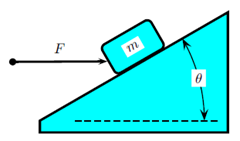# Problem: A block of mass m is on a frictionless plane inclined at θ with the horizontal and is pushed by a horizontal force F at a constant velocity up the plane. The acceleration of gravity is g . What is the magnitude of the normal force N the plane exerts on the block? 1. N = mg cos θ 2. N =F/ sin θ 3. N = F 4. N = mg 5. N = F tan θ 6. N = F sin θ 7. N =mg/ sin θ 8. N = mg tan θ 9. N =F/ cos θ 10. N = mg sin θ

🤓 Based on our data, we think this question is relevant for Professor Yuan's class at UCF.

###### Problem Details

A block of mass m is on a frictionless plane inclined at θ with the horizontal and is pushed by a horizontal force F at a constant velocity up the plane. The acceleration of gravity is g . What is the magnitude of the normal force N the plane exerts on the block?

1. N = mg cos θ

2. N =F/ sin θ

3. N = F

4. N = mg

5. N = F tan θ

6. N = F sin θ

7. N =mg/ sin θ

8. N = mg tan θ

9. N =F/ cos θ

10. N = mg sin θ